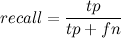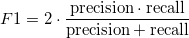# PostgreSQL User-Defined Aggregates

Aggregate functions compute a single result from a set of input values. Like sum() or count() they operate on one or more columns and multiple rows and output a single result.

In PostgreSQL is quite easy to create a custom aggregate function, the ingredients are:

• Create an aggregate function
• Create a state update function
• optional: create a final function

For example, if we want to implement the avg function we can write:

CREATE AGGREGATE avg (float8)
(
sfunc = float8_accum, -- {_count+=1, _sum+=value}
stype = float8[],
finalfunc = float8_avg, -- _sum / _count
initcond = '{0,0}'
);


Now we need a bit more complex aggregate function to write…

Suppose we need to compute some classification performance, we have a list of boolean couples like:

(actual, forecast) (actual, forecast) ...

Where actual is the true value and forecast is the output of some predictor.

Given a list of such boolean values is possible to define 4 quantities:

• true_positives = count of actual positives that are predicted as positives
• false_positives = count of actual negatives that are predicted as positives (mistakes)
• false_negatives = count of actual negatives that are predicted as positives (mistakes)
• true_negatives = count of actual negatives that are predicted as negatives

The matrix made by these 4 numbers is said confusion matrix and is a common synthetic representation of the informations about the performance of the used predictor.

Suppose we have this actual data:

 actual = 1 1 1 0 0 1 0 1 0 1 1

A naive predictor could be a majority voter (1s=7, 0s=4):

forecast = 1 1 1 1 1 1 1 1 1 1 1

The confusion matrix for this data and this predictor is:

tp=7 | fp=4 fn=0 | tn=0

Suppose we have some better predictor that gives us this forecast:

forecast = 1 1 1 0 0 0 1 1 1 1 1

This time the confusion matrix is:

tp=6 | fp=2 fn=1 | tn=2

As you can see the confusion matrix is compact, but there are still 4 numbers and it’s not easy to chose the best predictor looking at a matrix!

We can define two quantities, precision is the number of true positives over the number of predicted as positive, recall is the number of true positives over the number of actual positives.The F1 score is simply the harmonic mean of these two quantities:Now we have all the ingredients to build our aggregate function:

The state update function is the confusion_matrix, it accepts the current status and the two values needed to return the updated status.

-- Function: confusion_matrix(integer[], boolean, boolean)

CREATE OR REPLACE FUNCTION confusion_matrix(cmatrix integer[], actual boolean, forecast boolean)
RETURNS integer[] AS
$BODY$
SELECT ARRAY[
$1+($2 AND $3)::int, -- tp$1+((NOT $2) AND$3)::int,         -- fp
$1+($2 AND (NOT $3))::int, -- fn$1+((NOT $2) AND (NOT$3))::int    -- tn
]
$BODY$
LANGUAGE sql IMMUTABLE STRICT
COST 400;


The final function simply takes the current status and precess it:

-- Function: f1final(integer[])

CREATE OR REPLACE FUNCTION f1final(cmatrix integer[])
RETURNS real AS
$BODY$
SELECT CASE WHEN ("precision"($1) + recall($1)) = 0
THEN 'NaN'::float4
ELSE
(2.0 * ("precision"($1) * recall($1)) / ("precision"($1) + recall($1)))::float4
END
$BODY$
LANGUAGE sql IMMUTABLE STRICT
COST 100;


Here we have precision and recall functions (both valid as final functions too):

-- Function: "precision"(integer[])

CREATE OR REPLACE FUNCTION "precision"(cmatrix integer[])
RETURNS real AS
'SELECT (100.0*$1/($1 + $1))::float4' LANGUAGE sql IMMUTABLE STRICT COST 100; -- Function: recall(integer[]) CREATE OR REPLACE FUNCTION recall(cmatrix integer[]) RETURNS real AS 'SELECT (100.0*$1/($1 +$1))::float4'
LANGUAGE sql IMMUTABLE STRICT
COST 100;


And finally the real aggregate function that just keeps the pieces together:

-- Aggregate: f1

CREATE AGGREGATE f1(boolean, boolean) (
SFUNC=confusion_matrix,
STYPE=int4[],
FINALFUNC=f1final,
INITCOND='{0,0,0,0}'
);


Now, if we have a timeseries and we want to estimate the F1 score of the random walk predictor (a naive predictor that forecasts the last seen value), we can run a simple query:

select f1(ts1.value>0, ts2.value>0)
from timeseries as ts1, timeseries as ts2
where ts2.idx = ts1.idx - 1


And the results will be something like:

f1 ------ 76.423